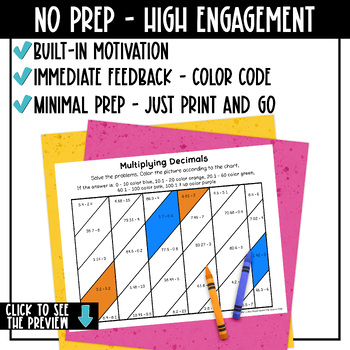# Decimal Operations Math Color Pages5th - 6th, Homeschool
Subjects
Standards
Resource Type
Formats Included
• PDF
•Activity
Pages
12 pages
Easel Activity Included
This resource includes a ready-to-use interactive activity students can complete on any device. Easel by TpT is free to use! Learn more.

### Description

Practice decimal operations with these printable math coloring pages. Students solve problems by adding, subtracting, multiplying, and dividing decimals. Then they use the code to color the picture. I've also added 2 sheets with mixed operations. Decimal Operations Color with Math includes 6 problem/color sheets and answer keys.

What's Included:

→ Subtracting Decimals (1 sheet)

→ Multiplying Decimals (1 sheet)

→ Dividing Decimals (1 sheet)

→ Decimal Operations 1 (1 sheet, add/subtract/multiply/divide)

→ Decimal Operations 2 (1 sheet, add/subtract/multiply/divide)

This resource is great for:

✧ Distance learning

✧ Independent work

✧ Math Centers, Math Stations

✧ At Home Learning, Homework

✧ Skill Practice or Review

Similar Resources & Skills:

◈ ◈ ◈ ◈ ◈ ◈ ◈ ◈ ◈ ◈ ◈ ◈ ◈ ◈ ◈ ◈ ◈ ◈ ◈ ◈ ◈ ◈ ◈ ◈ ◈ ◈ ◈ ◈ ◈ ◈ ◈ ◈ ◈ ◈ ◈ ◈ ◈ ◈

➯ Don't forget to leave feedback. You will receive TPT credits that can be used on future purchases!

Total Pages
12 pages
Included
Teaching Duration
Other
Report this Resource to TpT
Reported resources will be reviewed by our team. Report this resource to let us know if this resource violates TpT’s content guidelines.

### Standards

to see state-specific standards (only available in the US).
Fluently add, subtract, multiply, and divide multi-digit decimals using the standard algorithm for each operation.
Add, subtract, multiply, and divide decimals to hundredths, using concrete models or drawings and strategies based on place value, properties of operations, and/or the relationship between addition and subtraction; relate the strategy to a written method and explain the reasoning used.